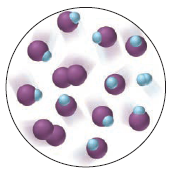# Problem: The molecular scene depicts a gaseous equilibrium mixture at 460°C for the reaction of H2 (blue) and I2 (purple) to form HI. The molecule represent 0.010 mol and the container volume is 1.0 L. (a) Is Kc &gt; 1, = 1, or &lt; 1?

###### FREE Expert Solution

For this problem, we are being asked to determine the Kc if it is > 1, =1 or < 1 using only the given diagram.

Recall that if Kc > 1, the equilibrium favors the formation of products.

If the Kc < 1, the equilibrium favors the formation of reactants.

93% (196 ratings)###### Problem Details

The molecular scene depicts a gaseous equilibrium mixture at 460°C for the reaction of H2 (blue) and I2 (purple) to form HI. The molecule represent 0.010 mol and the container volume is 1.0 L.

(a) Is Kc > 1, = 1, or < 1?Frequently Asked Questions

What scientific concept do you need to know in order to solve this problem?

Our tutors have indicated that to solve this problem you will need to apply the Chemical Equilibrium concept. You can view video lessons to learn Chemical Equilibrium. Or if you need more Chemical Equilibrium practice, you can also practice Chemical Equilibrium practice problems.

What professor is this problem relevant for?

Based on our data, we think this problem is relevant for Professor Sumner's class at UF.

What textbook is this problem found in?

Our data indicates that this problem or a close variation was asked in Chemistry: The Molecular Nature of Matter and Change - Silberberg 8th Edition. You can also practice Chemistry: The Molecular Nature of Matter and Change - Silberberg 8th Edition practice problems.